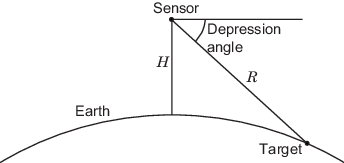# depressionang

Depression angle of surface target

Since R2021a

## Syntax

``depAng = depressionang(H,R)``
``depAng = depressionang(H,R,MODEL)``
``depAng = depressionang(H,R,MODEL,Re)``
``depAng = depressionang(___,TargetHeight=TGTHT)``

## Description

example

````depAng = depressionang(H,R)` returns the depression angle from the horizontal at an altitude of `R` meters to surface targets. The sensor is `H` meters above the surface. `R` is the range from the sensor to the surface targets. The computation assumes a curved earth model with an effective earth radius of approximately 4/3 times the actual earth radius.```
````depAng = depressionang(H,R,MODEL)` specifies the earth model used to compute the depression angle. `MODEL` is either `"Flat"` or `"Curved"`.```
````depAng = depressionang(H,R,MODEL,Re)` specifies the effective earth radius. Effective earth radius applies to a curved earth model. When `MODEL` is `"Flat"`, the function ignores `Re`.```
````depAng = depressionang(___,TargetHeight=TGTHT)` specifies the target height, `TGTHT` above the surface as either a scalar or a vector. If any combination of `H`, `R`, and `TGTHT` are vectors, then the dimensions must be equal. `r` must be greater than or equal to the absolute value of the difference of `ht` and `TGTHT`.```

## Examples

collapse all

Calculate the depression angle for a ground clutter patch that is 1.0 km away from a sensor. The sensor is located on a platform 300 m above the ground.

`depang = depressionang(300,1000)`
```depang = 17.4608 ```

## Input Arguments

collapse all

Height of the sensor above the surface in meters, specified as a scalar or a vector. If both `H` and `R` are nonscalar, they must have the same dimensions.

Data Types: `double`

Distance from the sensor to the surface target in meters, specified as a scalar or a vector. If both `H` and `R` are nonscalar, they must have the same dimensions. `R` must be between `H` and the horizon range determined by `TGTHT`.

Data Types: `double`

Earth model, specified as one of `"Curved"` or `"Flat"`.

Data Types: `char` | `string`

Effective earth radius in meters, specified as a positive scalar. You can use `effearthradius` to compute the effective radius. The function provides a default value approximately 4/3 times the actual earth radius

Example: `6.4e6`

Data Types: `double`

Target height above surface in meters, specified as a scalar or vector. If any combination of `H`, `R`, and `TGTHT` are vectors, then their sizes must be equal. `R` must be greater than or equal to the absolute value of the difference of `H` and `TGTHT`. A surface target has a `TGTHT` of zero.

Data Types: `double`

## Output Arguments

collapse all

Depression angle in degrees from the horizontal at the sensor altitude toward surface targets `R` meters from the sensor, returned as a scalar or a vector. If `depAng` is a vector, it has the same dimensions as the nonscalar inputs to `depressionang`.

collapse all

### Depression Angle

The depression angle is the angle between a horizontal line containing the sensor and the line from the sensor to a surface target.For the curved earth model with an effective earth radius of Re, the depression angle is:

`${\mathrm{sin}}^{-1}\left(\frac{{H}^{2}+2H{R}_{e}+{R}^{2}}{2R\left(H+{R}_{e}\right)}\right)$`

For the flat earth model, the depression angle is:

`${\mathrm{sin}}^{-1}\left(\frac{H}{R}\right)$`

 Long, Maurice W. Radar Reflectivity of Land and Sea, 3rd Ed. Boston: Artech House, 2001.

 Ward, J. "Space-Time Adaptive Processing for Airborne Radar Data Systems." Technical Report 1015, MIT Lincoln Laboratory, December 1994.